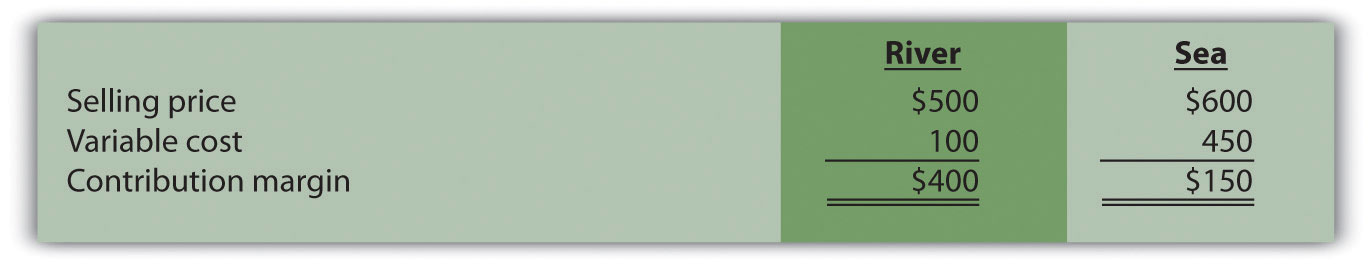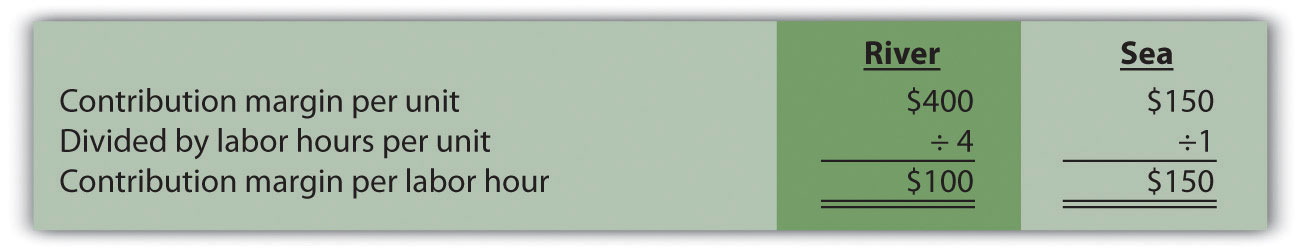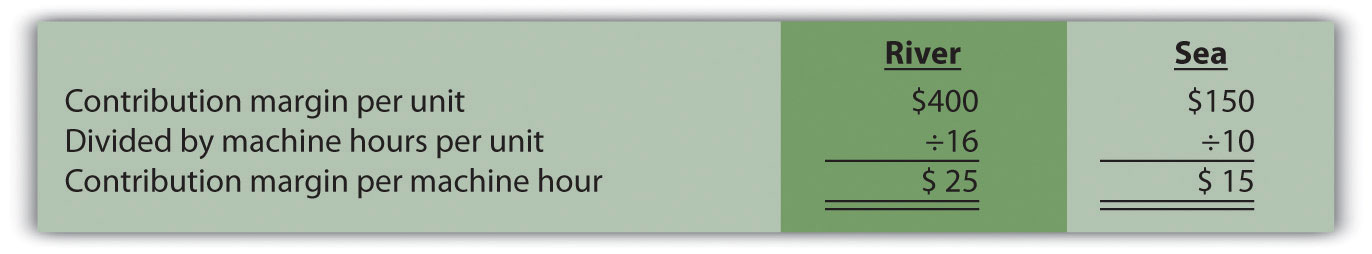## 6.5 Using a Contribution Margin When Faced with Resource Constraints

### Learning Objective

1. Use an alternative form of contribution margin when faced with a resource constraint.

Question: Many companies have limited resources in such areas as labor hours, machine hours, facilities, and materials. These constraints will likely affect a company’s ability to produce goods or provide services. When a company that produces multiple products faces a constraint, managers often calculate the contribution margin per unit of constraint in addition to the contribution margin per unit. The contribution margin per unit of constraintThe contribution margin per unit divided by the units of constrained resource required to produce one unit of product. is the contribution margin per unit divided by the units of constrained resource required to produce one unit of product. How is this measure used by managers to make decisions when faced with resource constraints?

Answer: Let’s examine the Kayaks-For-Fun example introduced earlier in the chapter. The company produces two kayak models, River and Sea. Based on the information shown, Kayaks-For-Fun would prefer to sell more of the River model because it has the highest contribution margin per unit.Kayaks-For-Fun has a total of 320 labor hours available each month. The specialized skills required to build the kayaks makes it difficult for management to find additional workers. Assume the River model requires 4 labor hours per unit and the Sea model requires 1 labor hour per unit (most of the variable cost for the Sea model is related to expensive materials required for production). Kayaks-For-Fun sells everything it produces. Given its labor hours constraint, the company would prefer to maximize the contribution margin per labor hour.Based on this information, Kayaks-For-Fun would prefer to sell the Sea model because it provides a contribution margin per labor hour of \$150 versus \$100 for the River model. The company would prefer only to make the Sea model, which would yield a total contribution margin of \$48,000 (= \$150 × 320 hours). If the River model were the only model produced, the total contribution margin to the company would be \$32,000 (= \$100 × 320 hours).

Analysis such as this often leads to further investigation. It may be that Kayaks-For-Fun can find additional labor to alleviate this resource constraint. Or perhaps the production process can be modified in a way that reduces the labor required to build the River model (e.g., through increased automation). Whatever the outcome, companies with limited resources are wise to calculate the contribution margin per unit of constrained resource.

### Key Takeaway

• Many organizations operate with limited resources in areas such as labor hours, machine hours, facilities, or materials. The contribution margin per unit of constraint is a helpful measure in determining how constrained resources should be utilized.

### Review Problem 6.6

This review problem is based on the information for Kayaks-For-Fun presented previously. Assume Kayaks-For-Fun found additional labor, thereby eliminating this resource constraint. However, the company now faces limited available machine hours. It has a total of 3,000 machine hours available each month. The River model requires 16 machine hours per unit, and the Sea model requires 10 machine hours per unit.

1. Calculate the contribution margin per unit of constrained resource for each model.
2. Which model would Kayaks-For-Fun prefer to sell to maximize overall company profit?

Solution to Review Problem 6.6

1.2. Kayaks-For-Fun would prefer to sell the River model because it provides a contribution margin per machine hour of \$25 compared to \$15 for the Sea model. If only the River model were sold, the total contribution margin would be \$75,000 (= \$25 × 3,000 machine hours). If only the Sea model were sold, the total contribution margin would be \$45,000 (= \$15 × 3,000 machine hours).